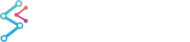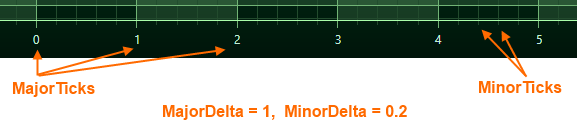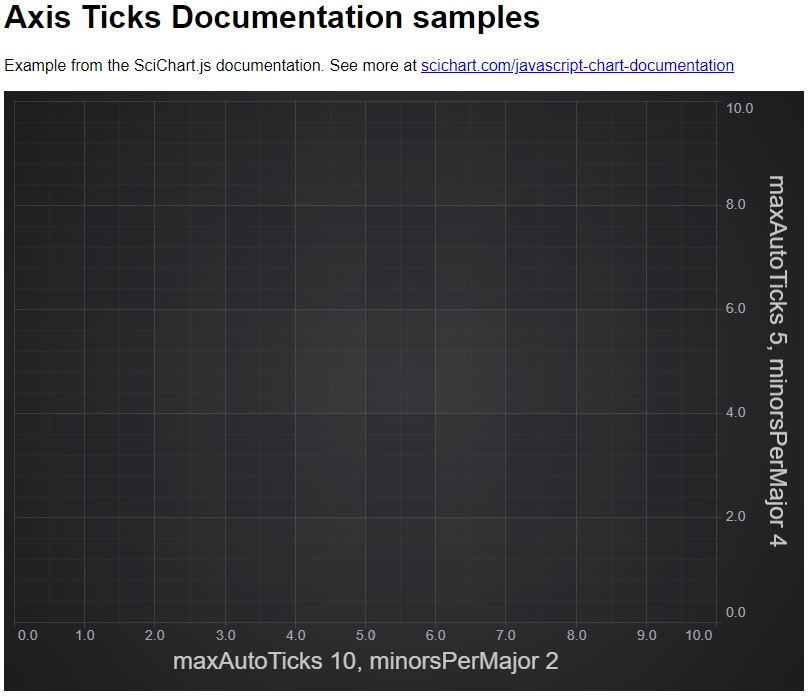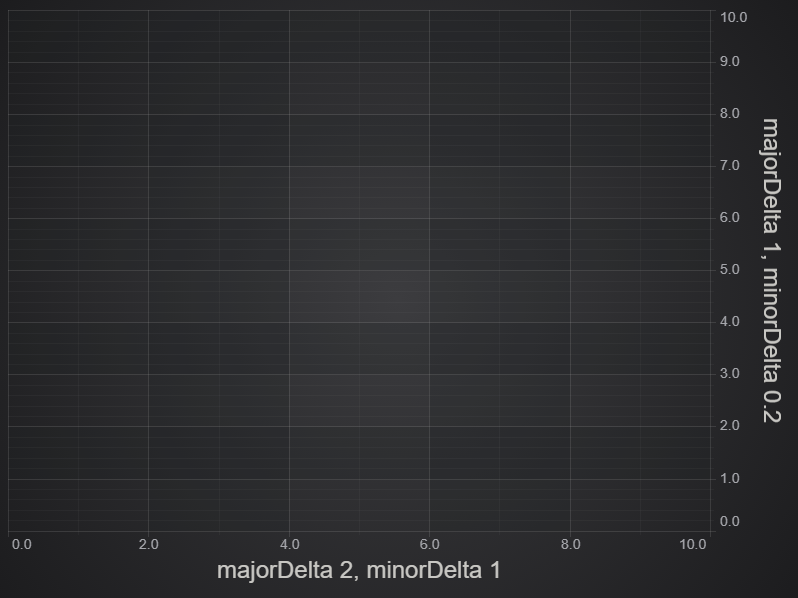﻿ Axis Ticks - Gridline and Label Spacing (Interval) | JavaScript Chart DocumentationSciChart.js JavaScript 2D Charts API > Axis APIs > Axis Ticks - Gridline and Label Spacing (Interval)
Axis Ticks - Gridline and Label Spacing (Interval)

## Axis Ticks, Labels and Grid Lines

In SciChart.js, the Ticks are small marks around the chart on an axis. There are Minor and Major Ticks, where Minor Ticks are placed in between Major ones. Axis Labels appears for every Major Tick, and Grid Lines correspond to Ticks on an axis.

In SciChart, axes are responsible not only for drawing Ticks and Labels, but also for the chart grid. So if you want to change the spacing of gridlines, labels or tick marks, you need to use the APIs to change Axis Tick spacing.## Automatic Gridline, Label or Tick Spacing

In SciChart.js, the difference between two Major Ticks is defined by axisCore.MajorDelta, and that between two minor Ticks - axisCore.MinorDelta

By default, MajorDelta and MinorDelta values are calculated automatically according to the VisibleRange and size of an axis in pixels. As zoom level changes, the MajorDelta, MinorDelta of every axis will be updated correspondingly, and the tick frequency may change.

If you want to enable Automatic Tick Spacing, set the autoTicks property to true, then set maxAutoTicks and minorsPerMajor properties:

Example Title
Copy Code
```import {SciChartSurface} from "scichart/Charting/Visuals/SciChartSurface";
import {NumericAxis} from "scichart/Charting/Visuals/Axis/NumericAxis";
export async function autoTicks(divId) {
const { wasmContext, sciChartSurface } = await SciChartSurface.create(divId);
const xAxis = new NumericAxis(wasmContext, { axisTitle: "maxAutoTicks 10, minorsPerMajor 2"});
// Default true, automatically calculate axis.MajorDelta, axis.MinorDelta
xAxis.autoTicks = true;
// This is a hint which defines the max number of major gridlines/labels visible at any one time.
// The actual number of gridlines may be lower than this depending on zoom level
xAxis.maxAutoTicks = 10;
// For every major gridline, this defines how many minor gridlines there are. Default is 5.
xAxis.minorsPerMajor = 2;
// Properties may also be set as constructor options
const yAxis = new NumericAxis(wasmContext, {
axisTitle: "maxAutoTicks 5, minorsPerMajor 4",
autoTicks: true,
maxAutoTicks: 5,
minorsPerMajor: 4
});
}
```## User-defined Gridline, Label and Tick Spacing (Method 1)

If you want control over the number of gridlines, labels and minor gridlines visible on the axis, set autoTicks to false and user the majorDelta and minorDelta properties. This is the first of two methods which will allow you to control gridline spacing.

Example Title
Copy Code
```import {SciChartSurface} from "scichart/Charting/Visuals/SciChartSurface";
import {NumericAxis} from "scichart/Charting/Visuals/Axis/NumericAxis";
export async function manualTicks(divId) {
const { wasmContext, sciChartSurface } = await SciChartSurface.create(divId);
const xAxis = new NumericAxis(wasmContext, { axisTitle: "majorDelta 2, minorDelta 1"});
// When autoTicks is false, you must specify majorDelta and minorDelta
xAxis.autoTicks = false;
// Have a major gridline every 2 units on the axis
xAxis.majorDelta = 2;
// Have a minor gridline every 1 unit on the axis
xAxis.minorDelta = 1;

// Properties may also be set as constructor options
const yAxis = new NumericAxis(wasmContext, {
axisTitle: "majorDelta 2, minorDelta 1",
autoTicks: false,
majorDelta: 1,
minorDelta: 0.2,
});
}
```This method could be dynamic if you combine with the event axis.visibleRangeChanged. However, if you want to have finer grained control over axis gridline, label or minor gridline spacing, then read on.

## Fine-grained control using TickProviders (Method 2)

If you want to have absolute control over the gridlines, labels and minor gridline spacing in SciChart.js, you can use the TickProvider API.

Every axis implementation has an axisCore.tickProvider property. This accepts a class which inherits TickProvider (TypeScript). Several classes are built-in such as NumericTickProvider which SciChart uses internally. You can create your own TickProvider if you want to have absolute control over the axis gridlines and label spacing. This API allows you to create some pretty advanced customisations with SciChart.js that are hard to achieve with other JavaScript chart providers.

Below here how our NumericTickProvider is implemented. The method getMajorTicks() returns an array of values where you want to place major gridlines and labels. The method getMinorTicks() returns an array of values where you want to place minor gridlines.

Example Title
Copy Code
```/**
* @summary The NumericTickProvider is a {@link TickProvider} implementation for Numeric 2D or 3D Axis.
* @description TickProviders are responsible for calculating the interval between major and minor gridlines, ticks and labels.
*
* The method {@link getMajorTicks} returns an array of major ticks (data-values values where SciChart will place labels and major gridlines.
* The method {@link getMinorTicks} returns an array of minor ticks (data-values values where SciChart will place minor gridlines.
* The method {@link isParamsValid} performs some sanity checks.
* The method {@link calculateTicks} performs the actual calculation
*
* Override these methods to create custom implementations of Tick intervals in SciChart
* @remarks
*/
export class NumericTickProvider extends TickProvider {
private readonly minDeltaValue: number = 1e-13;
private webAssemblyContext: TSciChart | TSciChart3D;
/**
* Creates an instance of a NumericTickProvider
* @param webAssemblyContext The {@link TSciChart | SciChart 2D WebAssembly Context} or {@link TSciChart | SciChart 3D WebAssembly Context}
* containing native methods and access to our WebGL2 WebAssembly Rendering Engine
*/
constructor(webAssemblyContext: TSciChart | TSciChart3D) {
super();
this.webAssemblyContext = webAssemblyContext;
}
/**
* @inheritDoc
*/
public getMinorTicks(minorDelta: number, majorDelta: number, visibleRange: NumberRange): number[] {
const deltaRange = new NumberRange(minorDelta, majorDelta);
const tickRange = visibleRange;
if (!this.isParamsValid(tickRange, deltaRange)) {
return [];
}
return this.calculateTicks(tickRange, deltaRange.min, deltaRange.max);
}
/**
* @inheritDoc
*/
public getMajorTicks(minorDelta: number, majorDelta: number, visibleRange: NumberRange): number[] {
const deltaRange = new NumberRange(minorDelta, majorDelta);
const tickRange = visibleRange;
if (!this.isParamsValid(tickRange, deltaRange)) {
return [];
}
return this.calculateTicks(tickRange, deltaRange.max, deltaRange.max);
}
/**
* @summary Performs sanity checks to see if parameters are valid.
* @decription If this method returns false, then we should not process or compute major/minor gridlines, but instead should
* @param visibleRange The current {@link AxisCore.visibleRange} which is the minimum / maximum range visible on the Axis.
* @param deltaRange The current {@link AxisCore.minorDelta} and {@link AxisCore.majorDelta} which is the difference between minor
* and major gridlines requested by the {@link AxisCore | Axis}
*/
protected isParamsValid(visibleRange: NumberRange, deltaRange: NumberRange): boolean {
Guard.notNull(visibleRange, "visibleRange");
Guard.notNull(deltaRange, "deltaRange");
return (
isRealNumber(visibleRange.min) &&
isRealNumber(visibleRange.max) &&
isRealNumber(deltaRange.min) &&
isRealNumber(deltaRange.max)
);
}
/**
* @summary Performs the Numeric tick calculation
* @param visibleRange The current {@link AxisCore.visibleRange} which is the minimum / maximum range visible on the Axis.
* @param delta The delta we are calculating for (could be major or minor delta)
* @param majorDelta The current {@link AxisCore.majorDelta} which is the difference between major
* gridlines requested by the {@link AxisCore | Axis}
*/
protected calculateTicks(visibleRange: NumberRange, delta: number, majorDelta: number): number[] {
const results: number[] = [];
const min = visibleRange.min;
const max = visibleRange.max;
let current = min;
const calcMajorTicks = delta === majorDelta;
const numberUtil = this.webAssemblyContext.NumberUtil;
if (!numberUtil.IsDivisibleBy(current, delta)) {
current = numberUtil.RoundUp(current, delta);
}
const start = current;
let tickCount = 0;
while (current <= max) {
// TRUE if major ticks are calculated && Current is divisible by MajorDelta
// or if minor ticks are calculated && Current is NOT divisible by MajorDelta
if (!(numberUtil.IsDivisibleBy(current, majorDelta) !== calcMajorTicks)) {
results.push(current);
}
current = start + ++tickCount * delta;
}
return results;
}
}
```

The usage of a TickProvider is to create an instance of the class and apply to axisCore.tickProvider property as follows:

Example Title
Copy Code
```import {SciChartSurface} from "scichart/Charting/Visuals/SciChartSurface";
import {NumericAxis} from "scichart/Charting/Visuals/Axis/NumericAxis";
import {NumericTickProvider} from "scichart/Charting/Numerics/TickProviders/NumericTickProvider";
import {NumberRange} from "scichart/Core/NumberRange";
import {TSciChart} from "scichart/types/TSciChart";
// A custom TickProvider can be used to have full control over axis tick (gridline and label) interval spacing
// use this API if you want to create complex rules on gridline/intervals, or dynamically changing rules
class CustomTickProvider extends NumericTickProvider {
constructor(wasmContext: TSciChart) {
super(wasmContext);
}
getMinorTicks(minorDelta: number, majorDelta: number, visibleRange: NumberRange): number[] {
// Todo here: calculate your tick spacing based on axis minorDelta, majorDelta and visibleRange
// Note we do not return major ticks here, so minor ticks exclude the majors
return [0.2, 0.4, 0.6, 0.8,
1.2, 1.4, 1.6, 1.8,
2.2, 2.4, 2.6, 2.8, 3.0, 3.2, 3.4, 3.6, 3.8,
4.2, 4.4, 4.6, 4.8, 5.0, 5.2, 5.4, 5.6, 5.8, 6.2, 6.4, 6.6, 6.8, 7.0, 7.2, 7.4, 7.6, 7.8];
}
getMajorTicks(minorDelta: number, majorDelta: number, visibleRange: NumberRange): number[] {
// Todo here: calculate your tick spacing based on axis minorDelta, majorDelta and visibleRange
// Note we return the major tick intervals and label intervals here
return [0,1,2,4,8];
}
}
export async function tickProvider(divId: string) {
const { wasmContext, sciChartSurface } = await SciChartSurface.create(divId);
const xAxis = new NumericAxis(wasmContext, {
axisTitle: "Custom TickProvider",
visibleRange: new NumberRange(0, 8)
});
xAxis.tickProvider = new CustomTickProvider(wasmContext);
const yAxis = new NumericAxis(wasmContext, {
axisTitle: "Custom TickProvider",
visibleRange: new NumberRange(0, 8)
});
yAxis.tickProvider = new CustomTickProvider(wasmContext);